Published

# Power Wheel with Variable Speed and Traction Control

Modified Power Wheel toy with variable speed capabilities and traction control

IntermediateWork in progress10 hours49## Things used in this project

### Hardware components×1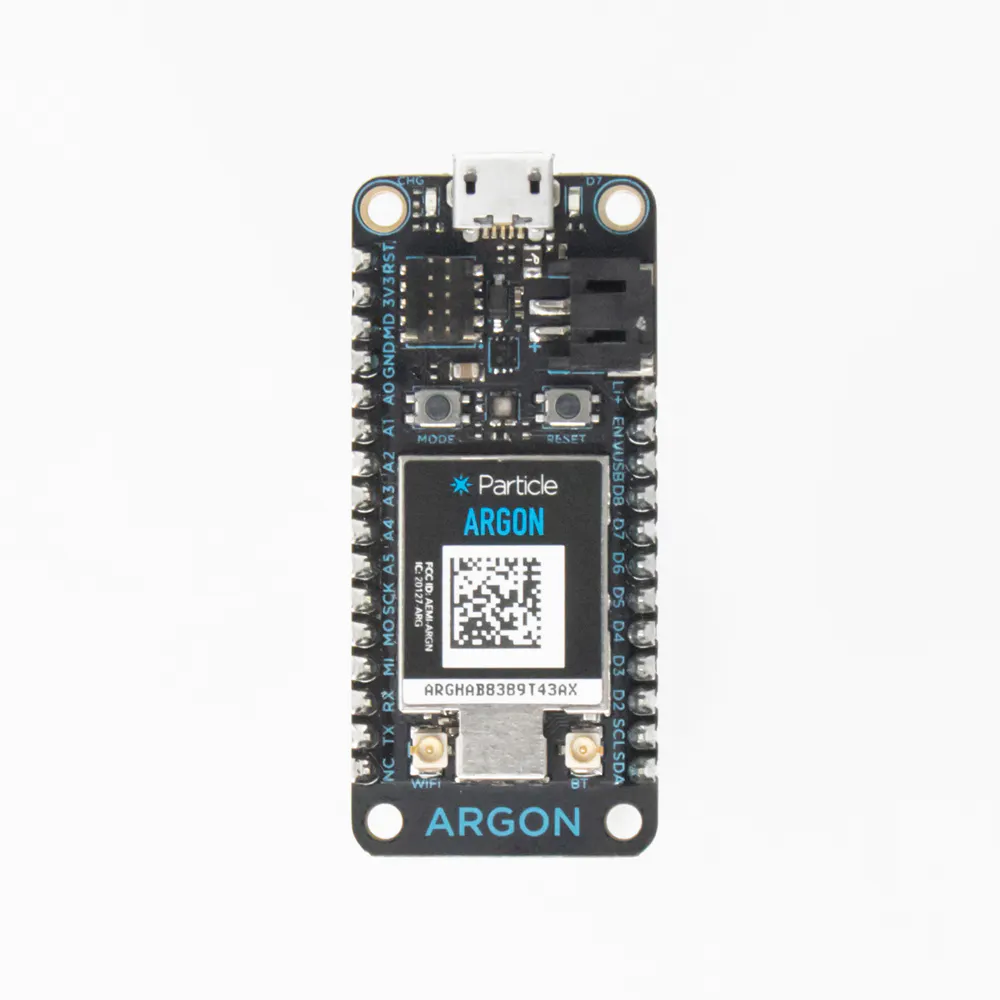Particle Argon
×1
 ES981 Solar Powerbank To supply power to Particle Argon
×1
 MAX471 3A Current Sensor Module Measures the current of the battery for current tracking.
×1Resistor 1k ohm For voltage divider to be able to measure voltage for voltage tracking.
×1Resistor 10k ohm For voltage divider to be able to measure voltage for voltage tracking.
×1
 BTS7960 43A High Power Motor Driver Motor driver to control speed and direction of motors.
×1
 MgcSTEM Variable Speed Pedal To give throttle control to control speed of car.
×1
 Craftsman 20V Power Wheel Power Wheel that was modified
×1

### Software apps and online servicesParticle Build Web IDE

### Hand tools and fabrication machinesSoldering iron (generic)Solder Flux, Soldering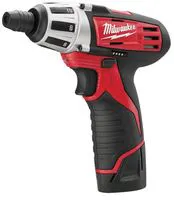Drill, Screwdriver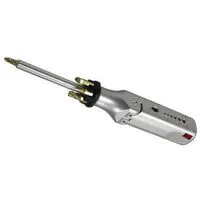Multitool, Screwdriver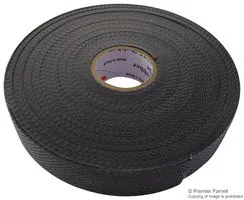Tape, Electrical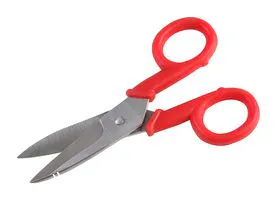Scissor, Electrician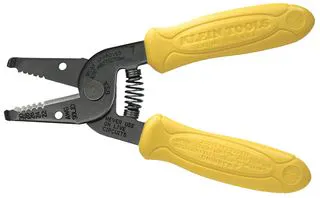Wire Stripper & Cutter, 18-10 AWG / 0.75-4mm² Capacity Wires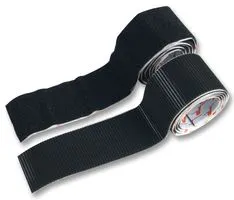Tape, Velcro® Stick On Tape/Strip

## Schematics

### Circuit Schematic

Circuit Schematic for power wheels project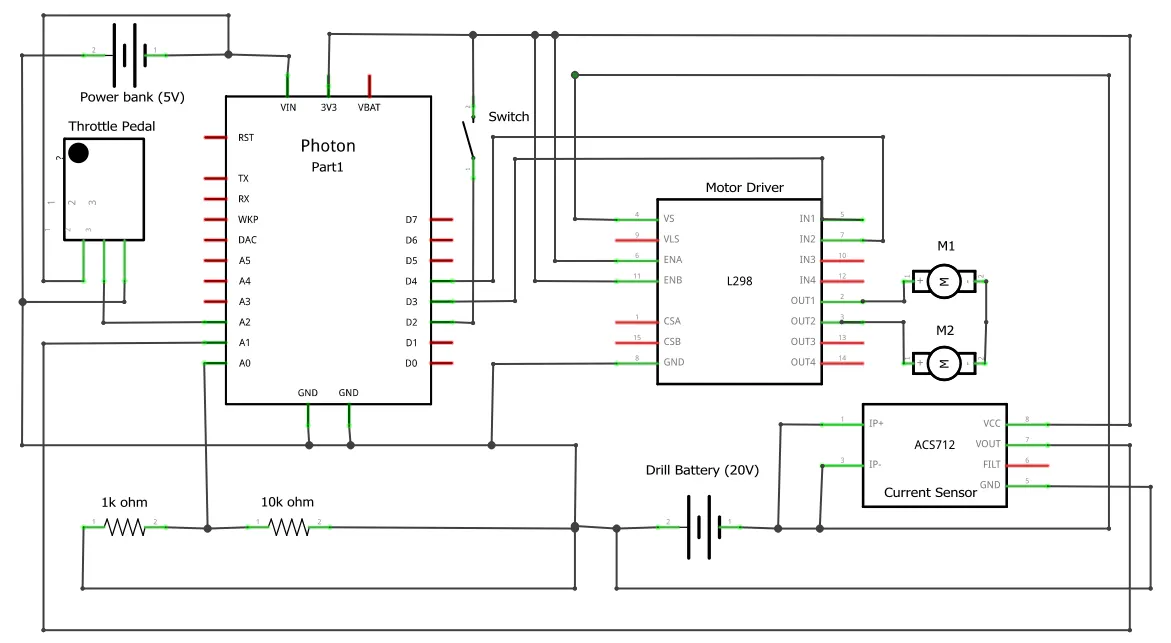## Code

### Power Wheel Code

C/C++
Controls an EV data logger to monitor current and voltage
```//Allows for code to operate if netwrok distruption occurs

const int VOLTAGE_PIN = A0;

const int CURRENT_PIN = A1;

// Defining Pin for pedal throttle control
const int PEDAL_PIN = A2;

// Initializing motor direction integer as 0
int motorDirection = 0;

// Setting digital pins for motor controller variable throttle and fwd/reverse

const int DIR_PIN = D2;
const int PWM_R = D3;
const int PWM_L = D4;
const int EN_A = D5;
const int EN_B = D6;

// Define the ratios for the voltage divider and current sensor calibration

const float VOLTAGE_RATIO = 0.657;

const float CURRENT_CALIBRATION = 0.04;

const float VOLTAGE_MULTI = 11; // Multiplies voltage to give an estimate of total voltage

const float Current_Corrector = 62.098; //Correct Current Readings

// Define the variables to hold the raw analog readings

int rawVoltage;

int rawCurrent;

// Defining variables to hold analog readings for motor control

int PedalPosition;

int motorSpeed;

//Defining variables for slew rate control based off throttle input
int THROTTLE_RATE =5;
int prevThrottle = 0;
int throttleOutput = 0;
int throttleInput=0;

// Define the variables to hold the filtered values

float filteredVoltage;

float filteredCurrent;

float totalVoltage;

float totalCurrent;

const unsigned long READ_INTERVAL = 20000;

// Define the time interval for outputting the filtered values (in milliseconds)

const unsigned long OUTPUT_INTERVAL = 10000;

// Define the filter coefficients for a first-order low-pass filter with a cutoff frequency of 5 Hz

const float FILTER_COEFFICIENT = 0.0183;

// Define the timer for outputting the filtered values

unsigned long outputTimer = 0;

void setup() {

// Initialize the serial communication

Serial.begin(9600);

// Setting pin modes for motor control connnections to the particle argon

pinMode(EN_A, OUTPUT);
pinMode(EN_B, OUTPUT);
pinMode(PWM_R, OUTPUT);
pinMode(PWM_L, OUTPUT);
pinMode(DIR_PIN, INPUT_PULLDOWN);

}

void loop() {

//Mapping pedal position to control motor position based on its position

motorSpeed = map(PedalPosition, 1500, 3100, 0, 255);
motorSpeed = constrain(motorSpeed, 0, 255);         //Contrains motor speed value to only return possible motor speed values

// Using direction pin to read if a voltage input is being sent into the pin to control forward/Reverse controls

// If/else statement to control motor based on direction and pedal input
if (motorDirection == HIGH)
{
digitalWrite(EN_A, HIGH);
digitalWrite(EN_B, LOW);
analogWrite(PWM_R, motorSpeed);
}
else
{
digitalWrite(EN_A, LOW);
digitalWrite(EN_B, HIGH);
analogWrite(PWM_L, motorSpeed);
}

// Slew rate traction control code
throttleInput = motorSpeed;
if (throttleInput > prevThrottle)
{
throttleOutput = min(throttleInput, prevThrottle + THROTTLE_RATE);
}
else {
throttleOutput = max(throttleInput, prevThrottle - THROTTLE_RATE);
}
prevThrottle = throttleOutput;

// Convert the raw values to voltages and current

float voltage = rawVoltage * 5.0 / 4095.0 * VOLTAGE_RATIO;

float current = (rawCurrent - 2047) * 5.0 / 4095.0 / CURRENT_CALIBRATION;

// Filter the values

filteredVoltage = FILTER_COEFFICIENT * voltage + (1 - FILTER_COEFFICIENT) * filteredVoltage;

filteredCurrent = FILTER_COEFFICIENT * current + (1 - FILTER_COEFFICIENT) * filteredCurrent;

totalVoltage = filteredVoltage * VOLTAGE_MULTI;

totalCurrent = (filteredCurrent + Current_Corrector) * (-1) ;

// Check if it's time to output the filtered values
if (millis() - outputTimer >= OUTPUT_INTERVAL) {

// Output the filtered values

Serial.print("Filtered voltage: ");

Serial.print(filteredVoltage);

Serial.print(" V, Filtered current: ");

Serial.print(filteredCurrent);

Serial.println(" A");

Serial.print("totalVoltage");

Serial.print("Throttle position");

// Reset the output timer

outputTimer = millis();

// Trigger the integration
Particle.publish("filtered voltage", String(filteredVoltage), PRIVATE);
Particle.publish("filtered current", String(totalCurrent), PRIVATE);
Particle.publish("total voltage", String(totalVoltage), PRIVATE);
Particle.publish("Throttle position", String(PedalPosition), PRIVATE);

}

unsigned long startTime = micros();

while (micros() - startTime < READ_INTERVAL) {

// Do nothing
}
}
```

## Credits

### Price Alphin

2 projects • 1 follower

### Braxton Bowen

2 projects • 1 follower

### Tanner Naumann

2 projects • 3 followers